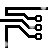## Computing Circuits

### By Robert J. Widlar

A circuit which performs both multiplication and division in this fashion.

In analog computation it is a relatively simple matter to perform such operations as addition, subtraction, integration and differentiation by incorporating the proper resistors and capacitors in the feedback circuit of an amplifier. Multiplication and division, however, are a bit more difficult. These operations are usually performed by taking the logarithms of the quantities, adding or subtracting as required and then taking the antilog.

At first glance, it might appear that obtaining the log of a voltage is difficult; but it has been shown6 that the emitter-base voltage of a silicon transistor follows the log of its collector current over as many as nine decades. This means that common transistors can be used to perform the log and antilog operations.Figure 1. Analog Multiplier/Divider

A circuit which performs both multiplication and division in this fashion is shown in Figure 1. It gives an output which is proportional to the product of two inputs divided by a third, and it is about the same complexity as a divider alone. The circuit consists of three log converters and an antilog generator. Log converters similar to these have been described elsewhere,7 but a brief description follows. Taking amplifier A1, a logging transistor, Q1, is inserted in the feedback loop such that its collector current is equal to the input voltage divided by the input resistor, R1. Hence, the emitter-base voltage of Q1 will vary as the log of the input voltage E1.

A2 is a similar amplifier operating with logging transistor, Q2. The emitter-base junctions of Q1 and Q2 are connected in series, adding the log voltages. The third log converter produces the log of E3. This is series-connected with the antilog transistor, Q4; and the combination is hooked in parallel with the output of the other two log convertors. Therefore, the emitter-base of Q4 will see the log of E3 subtracted from the sum of the logs of E1 and E2. Since the collector current of a transistor varies as the exponent of the emitter-base voltage, the collector current of Q4 will be proportional to the product of E1 and E2 divided by E3. This current is fed to the summing amplifier, A4, giving the desired output.

This circuit can give 1-percent accuracy for input voltages from 500 mV to 50V. To get this precision at lower input voltages, the offset of the amplifiers handling them must be individually balanced out. The zener diode, D4, increases the collector-base voltage across the logging transistors to improve high current operation. It is not needed, and is in fact undesirable, when these transistors are running at currents less than 0.3 mA. At currents above 0.3 mA, the lead resistances of the transistors can become important (0.25Ω is 1-percent at 1 mA) so the transistors should be installed with short leads and no sockets.

An important feature of this circuit is that its operation is independent of temperature because the scale factor change in the log converter with temperature is compensated by an equal change in the scale factor of the antilog generator. It is only required that Q1, Q2, Q3 and Q4 be at the same temperature. Dual transistors should be used and arranged as shown in the figure so that thermal mismatches between cans appear as inaccuracies in scale factor (0.3-percent/°C) rather than a balance error (8-percent/°C). R12 is a balance potentiometer which nulls out the offset voltages of all the logging transistors. It is adjusted by setting all input voltages equal to 2V and adjusting for a 2V output voltage.

The logging transistors provide a gain which is dependent on their operating level, which complicates frequency compensation. Resistors (R3, R6 and R7) are put in the amplifier output to limit the maximum loop gain, and the compensation capacitor is chosen to correspond with this gain.

Finally, clamp diodes D1 through D3, prevent exceeding the maximum reverse emitter-base voltage of the logging transistors with negative inputs.255408 December 2007